# Kruskal’s Minimum Spanning Tree Algorithm | Greedy Algo-2

• Difficulty Level : Hard
• Last Updated : 23 Jun, 2022

What is a Spanning Tree?

A Spanning tree is a subset to a connected graph G, where all the edges are connected, i.e, we can traverse to any edge from a particular edge with or without intermediates. Also, a spanning tree must not have any cycle in it. Thus we can say that if there are n vertices in a connected graph then the no. of edges that a spanning tree may have is n-1.

What is Minimum Spanning Tree?
Given a connected and undirected graph, a spanning tree of that graph is a subgraph that is a tree and connects all the vertices together. A single graph can have many different spanning trees. A minimum spanning tree (MST) or minimum weight spanning tree for a weighted, connected, undirected graph is a spanning tree with a weight less than or equal to the weight of every other spanning tree. The weight of a spanning tree is the sum of weights given to each edge of the spanning tree.
How many edges does a minimum spanning tree has?
A minimum spanning tree has (V – 1) edges where V is the number of vertices in the given graph.
What are the applications of the Minimum Spanning Tree?
See this for applications of MST.

Below are the steps for finding MST using Kruskal’s algorithm

1. Sort all the edges in non-decreasing order of their weight.
2. Pick the smallest edge. Check if it forms a cycle with the spanning tree formed so far. If cycle is not formed, include this edge. Else, discard it.
3. Repeat step#2 until there are (V-1) edges in the spanning tree.

Step #2 uses the Union-Find algorithm to detect cycles. So we recommend reading the following post as a prerequisite.
Union-Find Algorithm | Set 1 (Detect Cycle in a Graph)
Union-Find Algorithm | Set 2 (Union By Rank and Path Compression)
The algorithm is a Greedy Algorithm. The Greedy Choice is to pick the smallest weight edge that does not cause a cycle in the MST constructed so far. Let us understand it with an example: Consider the below input graph.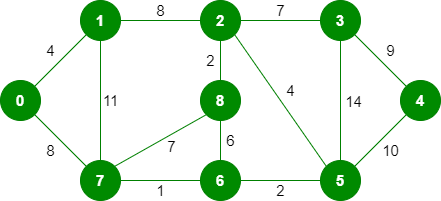The graph contains 9 vertices and 14 edges. So, the minimum spanning tree formed will be having (9 – 1) = 8 edges.

```After sorting:

Weight   Src    Dest
1         7      6
2         8      2
2         6      5
4         0      1
4         2      5
6         8      6
7         2      3
7         7      8
8         0      7
8         1      2
9         3      4
10        5      4
11        1      7
14        3      5```

Now pick all edges one by one from the sorted list of edges
1. Pick edge 7-6: No cycle is formed, include it.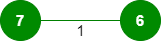2. Pick edge 8-2: No cycle is formed, include it.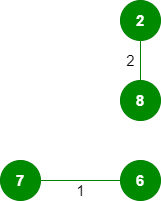3. Pick edge 6-5: No cycle is formed, include it.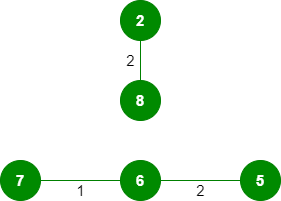4. Pick edge 0-1: No cycle is formed, include it.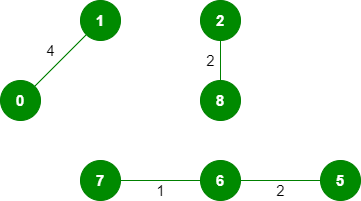5. Pick edge 2-5: No cycle is formed, include it.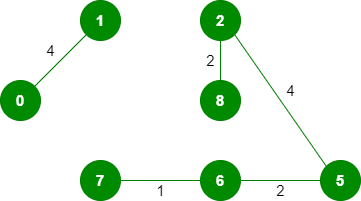6. Pick edge 8-6: Since including this edge results in the cycle, discard it.
7. Pick edge 2-3: No cycle is formed, include it.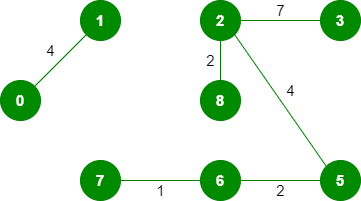8. Pick edge 7-8: Since including this edge results in the cycle, discard it.
9. Pick edge 0-7: No cycle is formed, include it.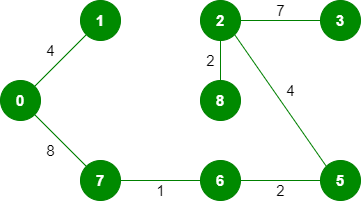10. Pick edge 1-2: Since including this edge results in the cycle, discard it.
11. Pick edge 3-4: No cycle is formed, include it.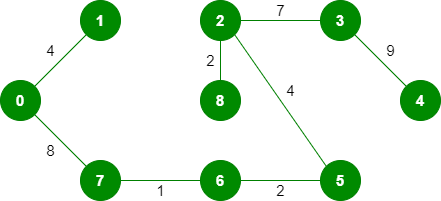Since the number of edges included equals (V – 1), the algorithm stops here.

Below is the implementation of the above idea:

## Java

 `// Java program for Kruskal's algorithm to``// find Minimum Spanning Tree of a given``//connected, undirected and  weighted graph``import` `java.util.*;``import` `java.lang.*;``import` `java.io.*;` `class` `Graph {``    ``// A class to represent a graph edge``    ``class` `Edge ``implements` `Comparable``    ``{``        ``int` `src, dest, weight;` `        ``// Comparator function used for``        ``// sorting edgesbased on their weight``        ``public` `int` `compareTo(Edge compareEdge)``        ``{``            ``return` `this``.weight - compareEdge.weight;``        ``}``    ``};` `    ``// A class to represent a subset for``    ``// union-find``    ``class` `subset``    ``{``        ``int` `parent, rank;``    ``};` `    ``int` `V, E; ``// V-> no. of vertices & E->no.of edges``    ``Edge edge[]; ``// collection of all edges` `    ``// Creates a graph with V vertices and E edges``    ``Graph(``int` `v, ``int` `e)``    ``{``        ``V = v;``        ``E = e;``        ``edge = ``new` `Edge[E];``        ``for` `(``int` `i = ``0``; i < e; ++i)``            ``edge[i] = ``new` `Edge();``    ``}` `    ``// A utility function to find set of an``    ``// element i (uses path compression technique)``    ``int` `find(subset subsets[], ``int` `i)``    ``{``        ``// find root and make root as parent of i``        ``// (path compression)``        ``if` `(subsets[i].parent != i)``            ``subsets[i].parent``                ``= find(subsets, subsets[i].parent);` `        ``return` `subsets[i].parent;``    ``}` `    ``// A function that does union of two sets``    ``// of x and y (uses union by rank)``    ``void` `Union(subset subsets[], ``int` `x, ``int` `y)``    ``{``        ``int` `xroot = find(subsets, x);``        ``int` `yroot = find(subsets, y);` `        ``// Attach smaller rank tree under root``        ``// of high rank tree (Union by Rank)``        ``if` `(subsets[xroot].rank``            ``< subsets[yroot].rank)``            ``subsets[xroot].parent = yroot;``        ``else` `if` `(subsets[xroot].rank``                 ``> subsets[yroot].rank)``            ``subsets[yroot].parent = xroot;` `        ``// If ranks are same, then make one as``        ``// root and increment its rank by one``        ``else` `{``            ``subsets[yroot].parent = xroot;``            ``subsets[xroot].rank++;``        ``}``    ``}` `    ``// The main function to construct MST using Kruskal's``    ``// algorithm``    ``void` `KruskalMST()``    ``{``        ``// This will store the resultant MST``        ``Edge result[] = ``new` `Edge[V];``      ` `        ``// An index variable, used for result[]``        ``int` `e = ``0``;``      ` `        ``// An index variable, used for sorted edges``        ``int` `i = ``0``;``        ``for` `(i = ``0``; i < V; ++i)``            ``result[i] = ``new` `Edge();` `        ``// Step 1:  Sort all the edges in non-decreasing``        ``// order of their weight.  If we are not allowed to``        ``// change the given graph, we can create a copy of``        ``// array of edges``        ``Arrays.sort(edge);` `        ``// Allocate memory for creating V subsets``        ``subset subsets[] = ``new` `subset[V];``        ``for` `(i = ``0``; i < V; ++i)``            ``subsets[i] = ``new` `subset();` `        ``// Create V subsets with single elements``        ``for` `(``int` `v = ``0``; v < V; ++v)``        ``{``            ``subsets[v].parent = v;``            ``subsets[v].rank = ``0``;``        ``}` `        ``i = ``0``; ``// Index used to pick next edge` `        ``// Number of edges to be taken is equal to V-1``        ``while` `(e < V - ``1``)``        ``{``            ``// Step 2: Pick the smallest edge. And increment``            ``// the index for next iteration``            ``Edge next_edge = edge[i++];` `            ``int` `x = find(subsets, next_edge.src);``            ``int` `y = find(subsets, next_edge.dest);` `            ``// If including this edge doesn't cause cycle,``            ``// include it in result and increment the index``            ``// of result for next edge``            ``if` `(x != y) {``                ``result[e++] = next_edge;``                ``Union(subsets, x, y);``            ``}``            ``// Else discard the next_edge``        ``}` `        ``// print the contents of result[] to display``        ``// the built MST``        ``System.out.println(``"Following are the edges in "``                           ``+ ``"the constructed MST"``);``        ``int` `minimumCost = ``0``;``        ``for` `(i = ``0``; i < e; ++i)``        ``{``            ``System.out.println(result[i].src + ``" -- "``                               ``+ result[i].dest``                               ``+ ``" == "` `+ result[i].weight);``            ``minimumCost += result[i].weight;``        ``}``        ``System.out.println(``"Minimum Cost Spanning Tree "``                           ``+ minimumCost);``    ``}` `    ``// Driver Code``    ``public` `static` `void` `main(String[] args)``    ``{` `        ``/* Let us create following weighted graph``                 ``10``            ``0--------1``            ``|  \     |``           ``6|   5\   |15``            ``|      \ |``            ``2--------3``                ``4       */``        ``int` `V = ``4``; ``// Number of vertices in graph``        ``int` `E = ``5``; ``// Number of edges in graph``        ``Graph graph = ``new` `Graph(V, E);` `        ``// add edge 0-1``        ``graph.edge[``0``].src = ``0``;``        ``graph.edge[``0``].dest = ``1``;``        ``graph.edge[``0``].weight = ``10``;` `        ``// add edge 0-2``        ``graph.edge[``1``].src = ``0``;``        ``graph.edge[``1``].dest = ``2``;``        ``graph.edge[``1``].weight = ``6``;` `        ``// add edge 0-3``        ``graph.edge[``2``].src = ``0``;``        ``graph.edge[``2``].dest = ``3``;``        ``graph.edge[``2``].weight = ``5``;` `        ``// add edge 1-3``        ``graph.edge[``3``].src = ``1``;``        ``graph.edge[``3``].dest = ``3``;``        ``graph.edge[``3``].weight = ``15``;` `        ``// add edge 2-3``        ``graph.edge[``4``].src = ``2``;``        ``graph.edge[``4``].dest = ``3``;``        ``graph.edge[``4``].weight = ``4``;` `        ``// Function call``        ``graph.KruskalMST();``    ``}``}``// This code is contributed by Aakash Hasija`

## Python3

 `# Python program for Kruskal's algorithm to find``# Minimum Spanning Tree of a given connected,``# undirected and weighted graph` `from` `collections ``import` `defaultdict` `# Class to represent a graph`  `class` `Graph:` `    ``def` `__init__(``self``, vertices):``        ``self``.V ``=` `vertices  ``# No. of vertices``        ``self``.graph ``=` `[]  ``# default dictionary``        ``# to store graph` `    ``# function to add an edge to graph``    ``def` `addEdge(``self``, u, v, w):``        ``self``.graph.append([u, v, w])` `    ``# A utility function to find set of an element i``    ``# (uses path compression technique)``    ``def` `find(``self``, parent, i):``        ``if` `parent[i] ``=``=` `i:``            ``return` `i``        ``return` `self``.find(parent, parent[i])` `    ``# A function that does union of two sets of x and y``    ``# (uses union by rank)``    ``def` `union(``self``, parent, rank, x, y):``        ``xroot ``=` `self``.find(parent, x)``        ``yroot ``=` `self``.find(parent, y)` `        ``# Attach smaller rank tree under root of``        ``# high rank tree (Union by Rank)``        ``if` `rank[xroot] < rank[yroot]:``            ``parent[xroot] ``=` `yroot``        ``elif` `rank[xroot] > rank[yroot]:``            ``parent[yroot] ``=` `xroot` `        ``# If ranks are same, then make one as root``        ``# and increment its rank by one``        ``else``:``            ``parent[yroot] ``=` `xroot``            ``rank[xroot] ``+``=` `1` `    ``# The main function to construct MST using Kruskal's``        ``# algorithm``    ``def` `KruskalMST(``self``):` `        ``result ``=` `[]  ``# This will store the resultant MST``        ` `        ``# An index variable, used for sorted edges``        ``i ``=` `0``        ` `        ``# An index variable, used for result[]``        ``e ``=` `0` `        ``# Step 1:  Sort all the edges in``        ``# non-decreasing order of their``        ``# weight.  If we are not allowed to change the``        ``# given graph, we can create a copy of graph``        ``self``.graph ``=` `sorted``(``self``.graph,``                            ``key``=``lambda` `item: item[``2``])` `        ``parent ``=` `[]``        ``rank ``=` `[]` `        ``# Create V subsets with single elements``        ``for` `node ``in` `range``(``self``.V):``            ``parent.append(node)``            ``rank.append(``0``)` `        ``# Number of edges to be taken is equal to V-1``        ``while` `e < ``self``.V ``-` `1``:` `            ``# Step 2: Pick the smallest edge and increment``            ``# the index for next iteration``            ``u, v, w ``=` `self``.graph[i]``            ``i ``=` `i ``+` `1``            ``x ``=` `self``.find(parent, u)``            ``y ``=` `self``.find(parent, v)` `            ``# If including this edge doesn't``            ``#  cause cycle, include it in result``            ``#  and increment the indexof result``            ``# for next edge``            ``if` `x !``=` `y:``                ``e ``=` `e ``+` `1``                ``result.append([u, v, w])``                ``self``.union(parent, rank, x, y)``            ``# Else discard the edge` `        ``minimumCost ``=` `0``        ``print` `(``"Edges in the constructed MST"``)``        ``for` `u, v, weight ``in` `result:``            ``minimumCost ``+``=` `weight``            ``print``(``"%d -- %d == %d"` `%` `(u, v, weight))``        ``print``(``"Minimum Spanning Tree"` `, minimumCost)` `# Driver code``g ``=` `Graph(``4``)``g.addEdge(``0``, ``1``, ``10``)``g.addEdge(``0``, ``2``, ``6``)``g.addEdge(``0``, ``3``, ``5``)``g.addEdge(``1``, ``3``, ``15``)``g.addEdge(``2``, ``3``, ``4``)` `# Function call``g.KruskalMST()` `# This code is contributed by Neelam Yadav`

## C#

 `// C# Code for above approach``using` `System;` `class` `Graph {` `    ``// A class to represent a graph edge``    ``class` `Edge : IComparable {``        ``public` `int` `src, dest, weight;` `        ``// Comparator function used for sorting edges``        ``// based on their weight``        ``public` `int` `CompareTo(Edge compareEdge)``        ``{``            ``return` `this``.weight``                   ``- compareEdge.weight;``        ``}``    ``}` `    ``// A class to represent``    ``// a subset for union-find``    ``public` `class` `subset``    ``{``        ``public` `int` `parent, rank;``    ``};` `    ``int` `V, E; ``// V-> no. of vertices & E->no.of edges``    ``Edge[] edge; ``// collection of all edges` `    ``// Creates a graph with V vertices and E edges``    ``Graph(``int` `v, ``int` `e)``    ``{``        ``V = v;``        ``E = e;``        ``edge = ``new` `Edge[E];``        ``for` `(``int` `i = 0; i < e; ++i)``            ``edge[i] = ``new` `Edge();``    ``}` `    ``// A utility function to find set of an element i``    ``// (uses path compression technique)``    ``int` `find(subset[] subsets, ``int` `i)``    ``{``        ``// find root and make root as``        ``// parent of i (path compression)``        ``if` `(subsets[i].parent != i)``            ``subsets[i].parent``                ``= find(subsets, subsets[i].parent);` `        ``return` `subsets[i].parent;``    ``}` `    ``// A function that does union of``    ``// two sets of x and y (uses union by rank)``    ``void` `Union(subset[] subsets, ``int` `x, ``int` `y)``    ``{``        ``int` `xroot = find(subsets, x);``        ``int` `yroot = find(subsets, y);` `        ``// Attach smaller rank tree under root of``        ``// high rank tree (Union by Rank)``        ``if` `(subsets[xroot].rank < subsets[yroot].rank)``            ``subsets[xroot].parent = yroot;``        ``else` `if` `(subsets[xroot].rank > subsets[yroot].rank)``            ``subsets[yroot].parent = xroot;` `        ``// If ranks are same, then make one as root``        ``// and increment its rank by one``        ``else` `{``            ``subsets[yroot].parent = xroot;``            ``subsets[xroot].rank++;``        ``}``    ``}` `    ``// The main function to construct MST``    ``// using Kruskal's algorithm``    ``void` `KruskalMST()``    ``{``        ``// This will store the``        ``// resultant MST``        ``Edge[] result = ``new` `Edge[V];``        ``int` `e = 0; ``// An index variable, used for result[]``        ``int` `i``            ``= 0; ``// An index variable, used for sorted edges``        ``for` `(i = 0; i < V; ++i)``            ``result[i] = ``new` `Edge();` `        ``// Step 1: Sort all the edges in non-decreasing``        ``// order of their weight. If we are not allowed``        ``// to change the given graph, we can create``        ``// a copy of array of edges``        ``Array.Sort(edge);` `        ``// Allocate memory for creating V subsets``        ``subset[] subsets = ``new` `subset[V];``        ``for` `(i = 0; i < V; ++i)``            ``subsets[i] = ``new` `subset();` `        ``// Create V subsets with single elements``        ``for` `(``int` `v = 0; v < V; ++v) {``            ``subsets[v].parent = v;``            ``subsets[v].rank = 0;``        ``}` `        ``i = 0; ``// Index used to pick next edge` `        ``// Number of edges to be taken is equal to V-1``        ``while` `(e < V - 1)``        ``{``            ``// Step 2: Pick the smallest edge. And increment``            ``// the index for next iteration``            ``Edge next_edge = ``new` `Edge();``            ``next_edge = edge[i++];` `            ``int` `x = find(subsets, next_edge.src);``            ``int` `y = find(subsets, next_edge.dest);` `            ``// If including this edge doesn't cause cycle,``            ``// include it in result and increment the index``            ``// of result for next edge``            ``if` `(x != y) {``                ``result[e++] = next_edge;``                ``Union(subsets, x, y);``            ``}``            ``// Else discard the next_edge``        ``}` `        ``// print the contents of result[] to display``        ``// the built MST``        ``Console.WriteLine(``"Following are the edges in "``                          ``+ ``"the constructed MST"``);``      ` `        ``int` `minimumCost = 0``        ``for` `(i = 0; i < e; ++i)``        ``{``            ``Console.WriteLine(result[i].src + ``" -- "``                              ``+ result[i].dest``                              ``+ ``" == "` `+ result[i].weight);``          ``minimumCost += result[i].weight;``        ``}``      ` `        ``Console.WriteLine(``"Minimum Cost Spanning Tree"``                          ``+ minimumCost);``        ``Console.ReadLine();``    ``}` `    ``// Driver Code``    ``public` `static` `void` `Main(String[] args)``    ``{` `        ``/* Let us create following weighted graph``                ``10``            ``0--------1``            ``| \ |``        ``6| 5\ |15``            ``| \ |``            ``2--------3``                ``4 */``        ``int` `V = 4; ``// Number of vertices in graph``        ``int` `E = 5; ``// Number of edges in graph``        ``Graph graph = ``new` `Graph(V, E);` `        ``// add edge 0-1``        ``graph.edge.src = 0;``        ``graph.edge.dest = 1;``        ``graph.edge.weight = 10;` `        ``// add edge 0-2``        ``graph.edge.src = 0;``        ``graph.edge.dest = 2;``        ``graph.edge.weight = 6;` `        ``// add edge 0-3``        ``graph.edge.src = 0;``        ``graph.edge.dest = 3;``        ``graph.edge.weight = 5;` `        ``// add edge 1-3``        ``graph.edge.src = 1;``        ``graph.edge.dest = 3;``        ``graph.edge.weight = 15;` `        ``// add edge 2-3``        ``graph.edge.src = 2;``        ``graph.edge.dest = 3;``        ``graph.edge.weight = 4;` `        ``// Function call``        ``graph.KruskalMST();``    ``}``}` `// This code is contributed by Aakash Hasija`

## C++

 `#include ``using` `namespace` `std;``// DSU data structure``//  path compression + rank by union` `class` `DSU {``    ``int``* parent;``    ``int``* rank;` `public``:``    ``DSU(``int` `n)``    ``{``        ``parent = ``new` `int``[n];``        ``rank = ``new` `int``[n];` `        ``for` `(``int` `i = 0; i < n; i++) {``            ``parent[i] = -1;``            ``rank[i] = 1;``        ``}``    ``}` `    ``// Find function``    ``int` `find(``int` `i)``    ``{``        ``if` `(parent[i] == -1)``            ``return` `i;` `        ``return` `parent[i] = find(parent[i]);``    ``}``    ``// union function``    ``void` `unite(``int` `x, ``int` `y)``    ``{``        ``int` `s1 = find(x);``        ``int` `s2 = find(y);` `        ``if` `(s1 != s2) {``            ``if` `(rank[s1] < rank[s2]) {``                ``parent[s1] = s2;``                ``rank[s2] += rank[s1];``            ``}``            ``else` `{``                ``parent[s2] = s1;``                ``rank[s1] += rank[s2];``            ``}``        ``}``    ``}``};` `class` `Graph {``    ``vector > edgelist;``    ``int` `V;` `public``:``    ``Graph(``int` `V) { ``this``->V = V; }` `    ``void` `addEdge(``int` `x, ``int` `y, ``int` `w)``    ``{``        ``edgelist.push_back({ w, x, y });``    ``}` `    ``void` `kruskals_mst()``    ``{``        ``// 1. Sort all edges``        ``sort(edgelist.begin(), edgelist.end());` `        ``// Initialize the DSU``        ``DSU s(V);``        ``int` `ans = 0;``        ``cout << ``"Following are the edges in the "``                ``"constructed MST"``             ``<< endl;``        ``for` `(``auto` `edge : edgelist) {``            ``int` `w = edge;``            ``int` `x = edge;``            ``int` `y = edge;` `            ``// take that edge in MST if it does form a cycle``            ``if` `(s.find(x) != s.find(y)) {``                ``s.unite(x, y);``                ``ans += w;``                ``cout << x << ``" -- "` `<< y << ``" == "` `<< w``                     ``<< endl;``            ``}``        ``}``        ``cout << ``"Minimum Cost Spanning Tree: "` `<< ans;``    ``}``};``int` `main()``{``    ``/* Let us create following weighted graph``                   ``10``              ``0--------1``              ``|  \     |``             ``6|   5\   |15``              ``|      \ |``              ``2--------3``                  ``4       */``    ``Graph g(4);``    ``g.addEdge(0, 1, 10);``    ``g.addEdge(1, 3, 15);``    ``g.addEdge(2, 3, 4);``    ``g.addEdge(2, 0, 6);``    ``g.addEdge(0, 3, 5);` `    ``// int n, m;``    ``// cin >> n >> m;` `    ``// Graph g(n);``    ``// for (int i = 0; i < m; i++)``    ``// {``    ``//     int x, y, w;``    ``//     cin >> x >> y >> w;``    ``//     g.addEdge(x, y, w);``    ``// }` `    ``g.kruskals_mst();``    ``return` `0;``}`

Output

```Following are the edges in the constructed MST
2 -- 3 == 4
0 -- 3 == 5
0 -- 1 == 10
Minimum Cost Spanning Tree: 19```

Time Complexity: O(ElogE) or O(ElogV). Sorting of edges takes O(ELogE) time. After sorting, we iterate through all edges and apply the find-union algorithm. The find and union operations can take at most O(LogV) time. So overall complexity is O(ELogE + ELogV) time. The value of E can be at most O(V2), so O(LogV) is O(LogE) the same. Therefore, the overall time complexity is O(ElogE) or O(ElogV)

References:
http://www.ics.uci.edu/~eppstein/161/960206.html
http://en.wikipedia.org/wiki/Minimum_spanning_tree